##### Quantum Physics For Dummies, Revised EditionIn quantum physics, ket notation makes the math easier than it is in matrix form because you can take advantage of a few mathematical relationships. For example, here’s the so-called Schwarz inequality for state vectors: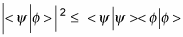This says that the square of the absolute value of the product of two state vectors,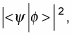is less than or equal to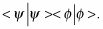This turns out to be the analog of the vector inequality: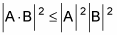So why is the Schwarz inequality so useful? It turns out that you can derive the Heisenberg uncertainty principle from it.

Other ket relationships can also simplify your calculations. For instance, two kets,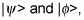are said to be orthogonal if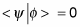And two kets are said to be orthonormal if they meet the following conditions: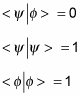This information is important for working with operators.